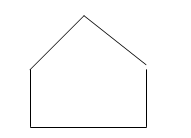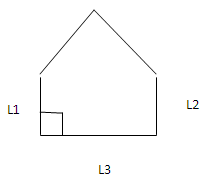# Learning Geometry

## Online Tutoring Is The Easiest, Most Cost-Effective Way For Students To Get The Help They Need Whenever They Need It.

People need to learn geometry because of the way it affects us daily. Learning geometric constructions helps us in understanding the world around us better. It hones one's thinking ability by using logical reasoning. Geometry facilitates the solution of problems from other fields since its principles are applicable to other disciplines. It can be used in a wide array of scientific and technical field. Geometry helps us to bring together both sides of our brain.

Example 1:Which statements can be used to describe the shape above?

A. The shape has neither parallel nor perpendicular line segments.
B. The shape has both parallel and perpendicular line segments.
C. The shape has parallel but not perpendicular line segments.
D. The shape has perpendicular but not parallel line segments.

Solution:

Perpendicular lines are lines in the same plane that intersect to form a right angle. Parallel lines are lines in the same plane that NEVER intersect.The picture above shows that L3 is perpendicular to L1 and L2. The picture also shows that L1 and L2 are parallel.

Therefore, the shape has both parallel and perpendicular line segments.

Example 2:
A circular table has a circumference of about 15 feet.
Develop an expression which can be used to find the approximate radius of the table?

Solution
Use the given approximation for the circumference of the table, as well as the formula for circumference.
Circumference of the circle C = 2 * pi * radius

Radius = 15 / (2 * pi)